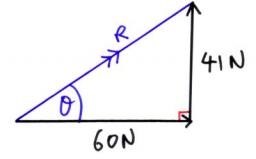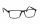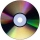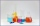# Forces

Determine the resultant of two perpendicular forces F1 = 560 N and second force of 25% smaller.

F =  700 N

### Step-by-step explanation:Did you find an error or inaccuracy? Feel free to write us. Thank you!Tips to related online calculators
Pythagorean theorem is the base for the right triangle calculator.

#### You need to know the following knowledge to solve this word math problem:

We encourage you to watch this tutorial video on this math problem:

## Related math problems and questions:

• ForcesIn point O acts three orthogonal forces: F1 = 20 N, F2 = 7 N, and F3 = 19 N. Determine the resultant of F and the angles between F and forces F1, F2, and F3.
• Forces on earth directionsA force of 60 N [North] and 80 N [East] is exerted on an object wigth 10 kg. What is the acceleration of the object?
• In the cinemaThere were 560 people in the cinema. 35% of them wore glasses. Of this number, 25% were women. How many men wore glasses?
• Two forcesTwo forces with magnitudes of 25 and 30 pounds act on an object at 10° and 100° angles. Find the direction and magnitude of the resultant force. Round to two decimal places in all intermediate steps and your final answer.
• Percents from percentHow much is 13% of 20% of 500 greater than 8% of 14% of 200?
• Sum of three numbersThe sum of three numbers from which second number is 20% smaller than the first number and the third number is 25% smaller than the second number is 96. Determine this numbers.
• SkisSkis, which cost € 800, became 25% more expensive before the season. After the season, they became cheaper again by 25%. How many euros did the skis cost after the discount?
• Content area and percentsDetermine what percentage is smaller cube surface, when the surface area of the wall decreases by 25%.
• Alcohol 2Two types of alcohol one 63% and second 75% give 20 liters of 69% alcohol. How many liters of each type are in the mixture?
• SolutionsHow much 60% solution and how much 35% solution is needed to create 100 l of 40% solution?
• The manufacturerThe manufacturer found that 3% of the plates produced had a malfunction. Of the compliant, 75% are first and 25% second. What is the probability of producing first and second class plates?
• Acid solutionBy adding 250 grams of a 96% sulfuric acid solution to its 3% solution, we changed its initial concentration to 25%. How many grams of 3% of the acid was used for dilution?
• ConcentrationHow many grams of 65% and 50% acid is to be mixed to give 240 g acid with a concentration of 60%?
• Alcohol mixingHow much 64% of the alcohol must be poured into 6 liters of 85% alcohol to produce 79% alcohol?
• Percentages above 100%What is 122% of 185? What is the meaning of percentages above 100%?
• CuSO4 mixtureHow many grams of solid CuSO4 we have to add to 450g of 15% CuSO4 solution to produce a 25% solution?
• GramsHow many grams of 5% solution do we need to add to 100g of 50% solution to get a 20% solution?